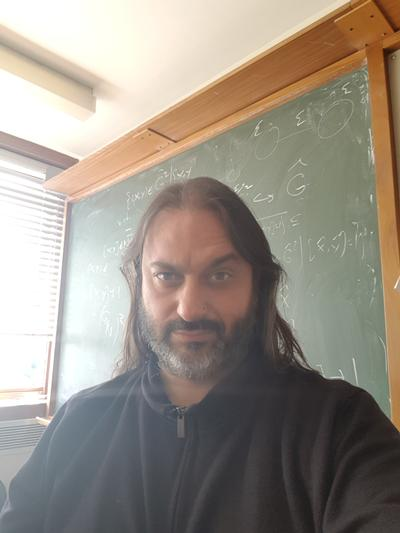The University of Southampton
Mathematical Sciences
Phone:
(023) 8059 5137
Email:
A.Martino@soton.ac.uk

Dr Armando Martino

Associate Professor of Pure MathematicsDr Armando Martino is an Associate Professor within Mathematical Sciences at the University of Southampton.

Background

Ramon y Cajal Postdoc, UPC, Barcelona

Postdoc, CRM, Barcelona

Temporary Lecturer, Cork

EPSRC Postdoc, Southampton

PhD in Mathematics, University of London, Queen Mary College

BA Mathematics, University of Oxford

Research interests

• Geometric group theory
Geometric Group Theory is the study of groups, that is the study of formal symmetry, in the context of their actions on suitably well behaved geometric objects. This incorporates such areas as the study of isometries of hyperbolic space, to the study of automorphisms of simplicial trees.
• Automorphisms of free groups
One of the basic objects in geometric group theory is that of free groups, which are groups that have no relations. These arise in topology as the fundamental groups of non-closed surfaces as well as in many other situations, such as in most linear groups, thanks to the Tits Alternative. An area of current interest is the study of automorphisms of free groups which are part of a grand analogy with mapping class groups of surfaces and arithmetic groups. In particular, the study of these groups focusses on their action on a finite dimensional contractible space known as Culler Vogtmann space. My recent interest has been to explore the geometry of this space, via the study of the Lipschitz metric.
• Group actions on trees
The classical theory of Bass-Serre gives detailed information on a group which has an action on a simplicial tree. More recently, there has been interest in the study of action on non-Archimedean trees, especially as this relates to equations in groups and the solution of the Tarski problem for free groups.
• Residual finiteness properties of groups
One of the most natural ways to study an infinite group is to understand it via its finite quotients. Residual finiteness is a property that a group has if one can distinguish elements of the group simply by consideration of its finite quotients. Conjugacy separability, a related property of interest to me, is the analogue of residual finiteness for conjugacy classes.

Pure Mathematics

Affiliate research group

Geometric Group Theory

Research project(s)

Profinite topology on non-positively curved groups

Questionnaire Organiser

Sort via:TypeorYear

Articles

Lecturer:
MATH3086, Galois Theory

Dr Armando Martino
Building 54, Mathematical Sciences, University of Southampton, Highfield, Southampton SO17 1BJ

Room Number: 54/7025

Facsimile: (023) 8059 5147